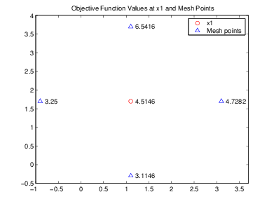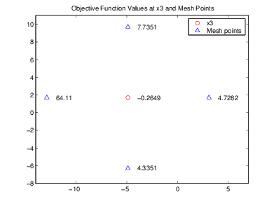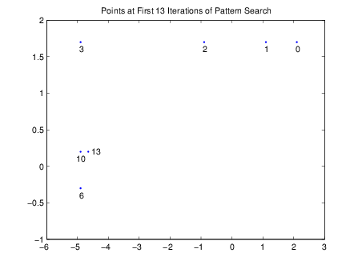## How Pattern Search Polling Works

### Context

`patternsearch` finds a sequence of points, `x0`, `x1`, `x2`, ... , that approach an optimal point. The value of the objective function either decreases or remains the same from each point in the sequence to the next. This section explains how pattern search works for the function described in Optimize Using the GPS Algorithm.

To simplify the explanation, this section describes how the generalized pattern search (GPS) works using the default maximal positive basis of 2N, with the `ScaleMesh` option set to `false`.

This section does not show how the `patternsearch` algorithm works with bounds or linear constraints. For bounds and linear constraints, `patternsearch` modifies poll points to be feasible at every iteration, meaning to satisfy all bounds and linear constraints.

This section does not encompass nonlinear constraints. To understand how `patternsearch` works with nonlinear constraints, see Nonlinear Constraint Solver Algorithm.

### Successful Polls

The pattern search begins at the initial point `x0` that you provide. In this example, `x0 = [2.1 1.7]`.

#### Iteration 1

At the first iteration, the mesh size is 1 and the GPS algorithm adds the pattern vectors to the initial point `x0 = [2.1 1.7]` to compute the following mesh points:

```[1 0] + x0 = [3.1 1.7] [0 1] + x0 = [2.1 2.7] [-1 0] + x0 = [1.1 1.7] [0 -1] + x0 = [2.1 0.7]```

The algorithm computes the objective function at the mesh points in the order shown above. The following figure shows the value of `ps_example` at the initial point and mesh points.The algorithm polls the mesh points by computing their objective function values until it finds one whose value is smaller than 4.6347, the value at `x0`. In this case, the first such point it finds is `[1.1 1.7]`, at which the value of the objective function is `4.5146`, so the poll at iteration 1 is successful. The algorithm sets the next point in the sequence equal to

`x1 = [1.1 1.7]`

Note

By default, the GPS pattern search algorithm stops the current iteration as soon as it finds a mesh point whose fitness value is smaller than that of the current point. Consequently, the algorithm might not poll all the mesh points. You can make the algorithm poll all the mesh points by setting the `UseCompletePoll` option to `true`.

#### Iteration 2

After a successful poll, the algorithm multiplies the current mesh size by 2, the default value of the `MeshExpansionFactor` options. Because the initial mesh size is 1, at the second iteration the mesh size is 2. The mesh at iteration 2 contains the following points:

```2*[1 0] + x1 = [3.1 1.7] 2*[0 1] + x1 = [1.1 3.7] 2*[-1 0] + x1 = [-0.9 1.7] 2*[0 -1] + x1 = [1.1 -0.3]```

The following figure shows the point `x1` and the mesh points, together with the corresponding values of `ps_example`.The algorithm polls the mesh points until it finds one whose value is smaller than 4.5146, the value at `x1`. The first such point it finds is `[-0.9 1.7]`, at which the value of the objective function is `3.25`, so the poll at iteration 2 is again successful. The algorithm sets the second point in the sequence equal to

`x2 = [-0.9 1.7]`

Because the poll is successful, the algorithm multiplies the current mesh size by 2 to get a mesh size of 4 at the third iteration.

### An Unsuccessful Poll

By the fourth iteration, the current point is

`x3 = [-4.9 1.7]`

and the mesh size is 8, so the mesh consists of the points

```8*[1 0] + x3 = [3.1 1.7] 8*[0 1] + x3 = [-4.9 9.7] 8*[-1 0] + x3 = [-12.9 1.7] 8*[0 -1] + x3 = [-4.9 -1.3]```

The following figure shows the mesh points and their objective function values.At this iteration, none of the mesh points has a smaller objective function value than the value at `x3`, so the poll is unsuccessful. In this case, the algorithm does not change the current point at the next iteration. That is,

`x4 = x3;`

At the next iteration, the algorithm multiplies the current mesh size by 0.5, the default value of the `MeshContractionFactor` option, so that the mesh size at the next iteration is 4. The algorithm then polls with a smaller mesh size.

### Successful and Unsuccessful Polls in MADS

Setting the `PollMethod` option to `'MADSPositiveBasis2N'` or `'MADSPositiveBasisNp1'` causes `patternsearch` to use both a different poll type and to react to polling differently than the other polling algorithms.

A MADS poll uses newly generated pseudorandom mesh vectors at each iteration. The vectors are randomly shuffled components from the columns of a random lower-triangular matrix. The components of the matrix have integer sizes up to 1/. In the poll, the mesh vectors are multiplied by the mesh size, so the poll points can be up to from the current point.

Unsuccessful polls contract the mesh by a factor of `4`, ignoring the `MeshContractionFactor` option. Similarly, successful polls expand the mesh by a factor of `4`, ignoring the `MeshExpansionFactor` option. The maximum mesh size is `1`, despite any setting of the `MaxMeshSize` option.

In addition, when there is a successful poll, `patternsearch` starts at the successful point and polls again. This extra poll uses the same mesh vectors, expanded by a factor of `4` while staying below size `1`. The extra poll looks again along the same directions that were just successful.

### Displaying the Results at Each Iteration

You can display the results of the pattern search at each iteration by setting the `Display` option to `'iter'`. This enables you to evaluate the progress of the pattern search and to make changes to `options` if necessary.

With this setting, the pattern search displays information about each iteration at the command line. The first four iterations are

```Iter f-count f(x) MeshSize Method 0 1 4.63474 1 1 4 4.51464 2 Successful Poll 2 7 3.25 4 Successful Poll 3 10 -0.264905 8 Successful Poll 4 14 -0.264905 4 Refine Mesh```

The entry `Successful Poll` below `Method` indicates that the current iteration was successful. For example, the poll at iteration 2 is successful. As a result, the objective function value of the point computed at iteration 2, displayed below `f(x)`, is less than the value at iteration 1.

At iteration 4, the entry `Refine Mesh` tells you that the poll is unsuccessful. As a result, the function value at iteration 4 remains unchanged from iteration 3.

By default, the pattern search doubles the mesh size after each successful poll and halves it after each unsuccessful poll.

### More Iterations

The pattern search performs 60 iterations before stopping. The following plot shows the points in the sequence computed in the first 13 iterations of the pattern search.The numbers below the points indicate the first iteration at which the algorithm finds the point. The plot only shows iteration numbers corresponding to successful polls, because the best point doesn't change after an unsuccessful poll. For example, the best point at iterations 4 and 5 is the same as at iteration 3.

### Poll Method

At each iteration, the pattern search polls the points in the current mesh—that is, it computes the objective function at the mesh points to see if there is one whose function value is less than the function value at the current point. How Pattern Search Polling Works provides an example of polling. You can specify the pattern that defines the mesh by the `PollMethod` option. The default pattern, `'GPSPositiveBasis2N'`, consists of the following 2N directions, where N is the number of independent variables for the objective function.

[1 0 0...0]
[0 1 0...0]
...
[0 0 0...1]
[–1 0 0...0]
[0 –1 0...0]
[0 0 0...–1].

For example, if the objective function has three independent variables, the `GPS Positive basis 2N`, consists of the following six vectors.

[1 0 0]
[0 1 0]
[0 0 1]
[–1 0 0]
[0 –1 0]
[0 0 –1].

Alternatively, you can set the `PollMethod` option to `'GPSPositiveBasisNp1'`, the pattern consisting of the following N + 1 directions.

[1 0 0...0]
[0 1 0...0]
...
[0 0 0...1]
[–1 –1 –1...–1].

For example, if objective function has three independent variables, the `'GPSPositiveBasisNp1'` consists of the following four vectors.

[1 0 0]
[0 1 0]
[0 0 1]
[–1 –1 –1].

A pattern search will sometimes run faster using `'GPSPositiveBasisNp1'` rather than the `'GPSPositiveBasis2N'` as the `PollMethod`, because the algorithm searches fewer points at each iteration. Although not being addressed in this example, the same is true when using the `'MADSPositiveBasisNp1'` over the `'MADSPositiveBasis2N'`, and similarly for GSS. For example, if you run a pattern search on the linearly constrained example in Constrained Minimization Using patternsearch and Optimize Live Editor Task, the algorithm performs 1588 function evaluations with `'GPSPositiveBasis2N'`, the default `PollMethod`, but only 877 function evaluations using `'GPSPositiveBasisNp1'`. For more detail, see Compare the Efficiency of Poll Options.

However, if the objective function has many local minima, using `'GPSPositiveBasis2N'` as the `PollMethod` might avoid finding a local minimum that is not the global minimum, because the search explores more points around the current point at each iteration.

### Complete Poll

By default, if the pattern search finds a mesh point that improves the value of the objective function, it stops the poll and sets that point as the current point for the next iteration. When this occurs, some mesh points might not get polled. Some of these unpolled points might have an objective function value that is even lower than the first one the pattern search finds.

For problems in which there are several local minima, it is sometimes preferable to make the pattern search poll all the mesh points at each iteration and choose the one with the best objective function value. This enables the pattern search to explore more points at each iteration and thereby potentially avoid a local minimum that is not the global minimum. Have the pattern search poll the entire mesh setting the `UseCompletePoll` option to `true`.

### Stopping Conditions for the Pattern Search

The algorithm stops when any of the following conditions occurs:

• The mesh size is less than the `MeshTolerance` option.

• The number of iterations performed by the algorithm reaches the value of the `MaxIterations` option.

• The total number of objective function evaluations performed by the algorithm reaches the value of the `MaxFunctionEvaluations` option.

• The time, in seconds, the algorithm runs until it reaches the value of the `MaxTime` option.

• After a successful poll, the distance between the point found in the previous two iterations and the mesh size are both less than the `StepTolerance` option.

• After a successful poll, the change in the objective function in the previous two iterations is less than the `FunctionTolerance` option and the mesh size is less than the `StepTolerance` option.

The `ConstraintTolerance` option is not used as stopping criterion. It determines the feasibility with respect to nonlinear constraints.

The MADS algorithm uses an additional parameter called the poll parameter, Δp, in the mesh size stopping criterion:

where Δm is the mesh size. The MADS stopping criterion is:

Δp ≤ `MeshTolerance`.

### Robustness of Pattern Search

The pattern search algorithm is robust in relation to objective function failures. This means `patternsearch` tolerates function evaluations resulting in `NaN`, `Inf`, or complex values. When the objective function at the initial point `x0` is a real, finite value, `patternsearch` treats poll point failures as if the objective function values are large, and ignores them.

For example, if all points in a poll evaluate to `NaN`, `patternsearch` considers the poll unsuccessful, shrinks the mesh, and reevaluates. If even one point in a poll evaluates to a smaller value than any seen yet, `patternsearch` considers the poll successful, and expands the mesh.

## SupportGet trial now